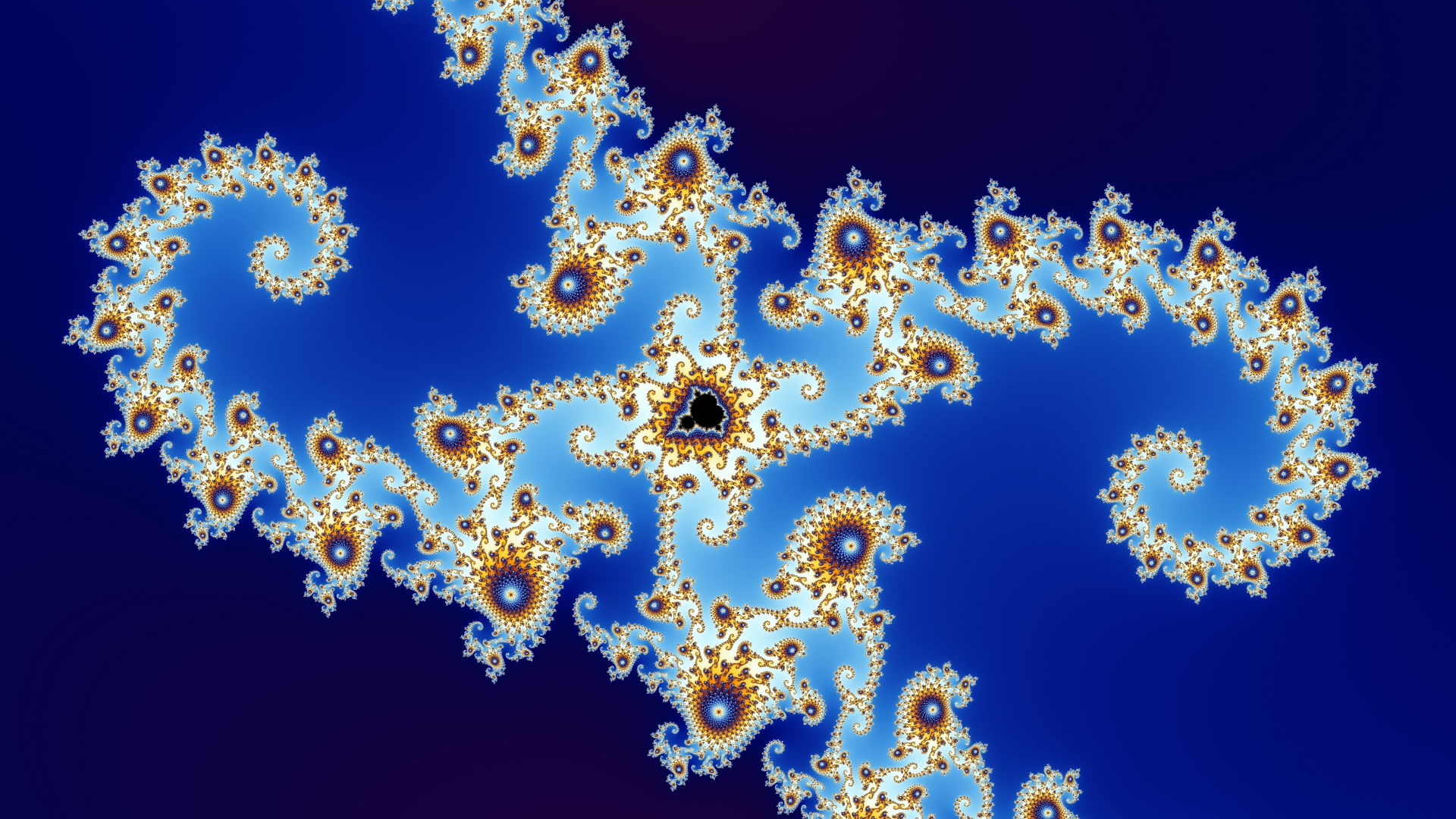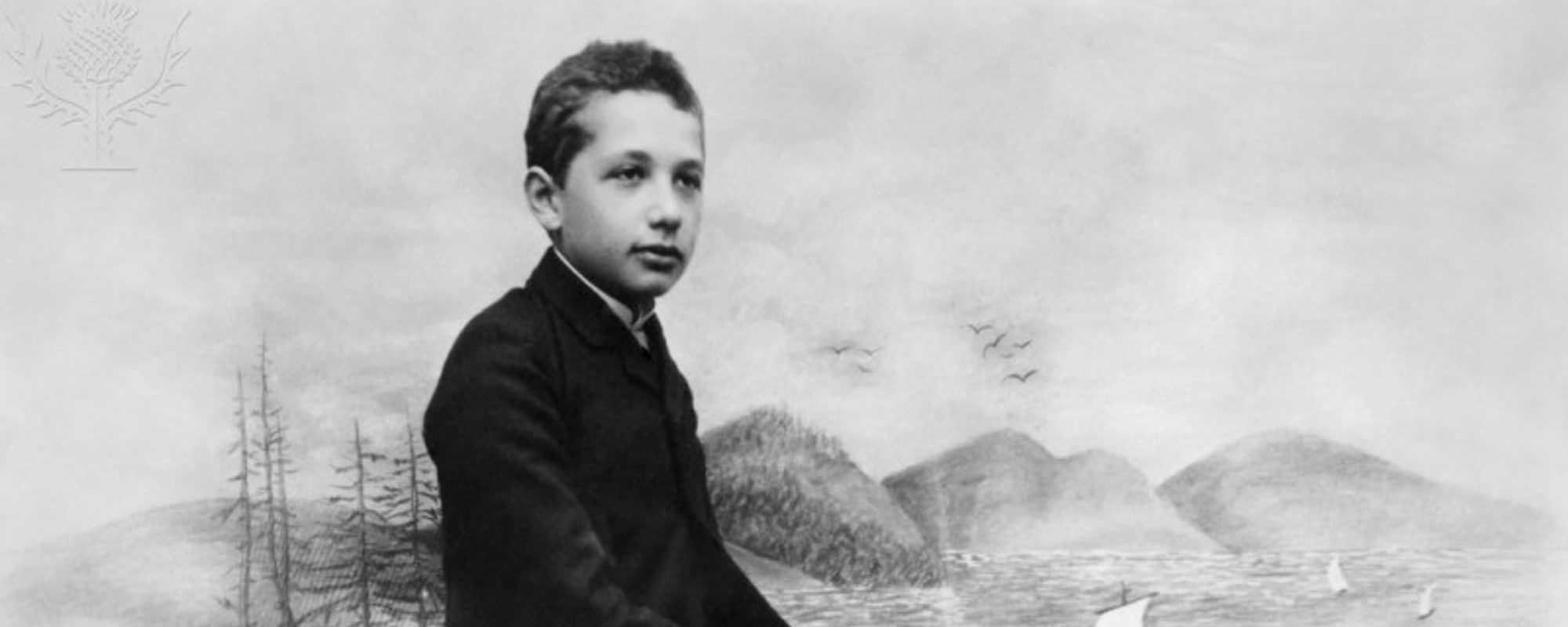# Complex numbers: an introduction

We will leave the domain of real numbers behind us and start exploring the plane of complex numbers. An introduction to a realm beyond imagination. Continue reading Complex numbers: an introduction# Heisenberg’s uncertainty principle

Heisenberg’s uncertainty principle is famous in quantum mechanics. However, it doesn’t have its roots in quantum mechanics. Let’s look at Fourier transform pairs. Continue reading Heisenberg’s uncertainty principle# Quantum entanglement: the EPR paradox and Bell’s Theorem

Einstein didn’t like that quantum entanglement seemed to imply information travelling faster than the speed of light. We discuss Bell’s Theorem. Continue reading Quantum entanglement: the EPR paradox and Bell’s Theorem# Quantum entanglement: non-locality and the state of a two-particle system

In this two-parter, we discuss quantum entanglement, non-locality, and some mathematics. In the next post, we discuss the EPR paradox and Bell’s Theorem. Continue reading Quantum entanglement: non-locality and the state of a two-particle system# Lab centrifuges and prime numbers

Lab centrifuges are crucial in e.g. coronavirus research. It’s vital the test tubes are balanced. There is an easy method to know if that’s possible. Continue reading Lab centrifuges and prime numbers# Finding the normal force in planar non-uniform circular motion using polar coordinates

We find an expression for the normal force on a mass which is in planar non-uniform circular motion using polar coordinates. Continue reading Finding the normal force in planar non-uniform circular motion using polar coordinates# Why do wet clothes dry?

We discuss the second law of thermodynamics, the notion of entropy, the statistical nature of the situation, and why wet clothes dry. Continue reading Why do wet clothes dry?# The formula that got Albert Einstein the Nobel Prize and should stop us getting sunburn all the time

Albert Einstein didn’t win the Nobel Prize with his famous formula from the special theory of relativity. What formula did he win the Prize with then? Continue reading The formula that got Albert Einstein the Nobel Prize and should stop us getting sunburn all the time# What is a spacetime interval?

Einstein and collaborators taught us that space and time are not fixed quantities. They can stretch and contract. They vary. There is one thing, though, that does not vary. It is the invariance of the spacetime interval. Continue reading What is a spacetime interval?# Deriving the Lorentz transformations from a rotation of frames of reference about their origin with real time Wick-rotated to imaginary time

Well-known for their central role in Einstein’s Special Relativity, the Lorentz transformations are derived from the rotation of two frames of reference in standard configuration while time is taken to be an imaginary unit of spacetime. This is rarely seen in the wild. Not many undergraduate textbooks or online texts show the details of the working. Hence, this article. Continue reading Deriving the Lorentz transformations from a rotation of frames of reference about their origin with real time Wick-rotated to imaginary time